# TARGET SBI EXAM 2018: REASONING –Blood relation – 1

Dear Bankersdaily Aspirants,

Hope , Your preparation for SBI Clerk & PO exam 2018 is good . Since SBI Clerk & PO exam is a Two level which will be conducted in an online mode, Competition will be higher for this SBI Clerk & PO Exam and the most important thing , Current affairs section will be there in this exam. So aspirants have to concentrate in Four sections namely Aptitude, Reasoning, English, Current Affairs.

The person with high speed and very good accuracy only can crack this exam to taste the success. The candidates will be selected on the basis of performance in this online exam test. So the candidate with consistent hard work and regular practice will crack the exam very easily.

Exam: SBI Clerk & PO Exam 2018

Topic: Blood relation – 1

Timing: 7 minutes

D.1-2) Study the following information carefully and answer the questions.

A is the father of B and son in law of E. F is the niece of R who is mother of Z. R is the sister in law of H who is grandmother of B. H is not a parent of A.

Q.1) If Z is married to K and he is a banker then how is K related to R?

a) Brother in law

b) Sister in law

c) Son in law

d) Daughter in law

e) None of these

Q.2) How is H related to A?

a) Father in law

b) Mother in law

c) Son in law

d) Daughter in law

e) None of these

D.3-4) Study the following information and answer the given questions.

P and R are married couple. S is the only sister of R who is the child of T. K is the son in law of T. S is related to K in a same way P is related to R.

Q.3) How is R related to P?

a) Can’t be determined

b) Either c or d

c) Husband

d) Wife

e) None of these

Q.4) If Q is the son of R then how is S related to Q?

a) Paternal aunt

b) Maternal aunt

c) Niece

d) Nephew

e) None of these

Q.5) X, Y, S, T, R, M and Q live in a same family. X and Y are sisters of M and they are married to R and T respectively. T is the son in law of S. Q is daughter in law of S then how is Q related to Y?

a) Brother in law

b) Sister in law

c) Uncle

d) Aunt

e) None of these

D.6-10) In the following questions, the symbols @, *, ?, ! and # are used with the follow in; meanings as illustrated below:

`P @ Q’ means `P is son of Q’.

`P # Q’ means ‘Q is father of P’.

`P ? Q’ means `Q is daughter of P’.

`P ! Q’ means `P is mother of Q’.

`P * Q’ means `P is brother of Q’.

Q.6) How is W related to K where the following expression is definitely true?

J @ K ! T * W ? X # Z.

a) Sister

b) Daughter

c) Son

d) Brother

e) None of these

Q.7) How is Z related to T where the following expression is definitely true?

A * D ! Z ? P @ K * T.

a) Sister in law

b) Daughter in law

c) Son in law

d) Brother in law

e) None of these

Q.8) Which of the following statement is false where the following expression is definitely true R * W * K ? L # M @ Q.

a) K is wife of M

b) R is brother in law of M

c) L is granddaughter of Q

d) M is mother of L

e) None of these

Q.9) In which of the following expressions shows that E is paternal aunt of C?

a) A!B?C#D@E

b) D#E*B@C?A

c) E!B@D@C?F

d) B*C#A@D?E

e) None of these

Q.10) Which of the following symbol should come in the place of _ in this expression ‘J@K_L_M*N#O*P’ that L is nephew of N and K is sister in law of N is definitely true?

a) @, @

b) *, *

c) !, @

d) @, !

e) None of these

D.1-2)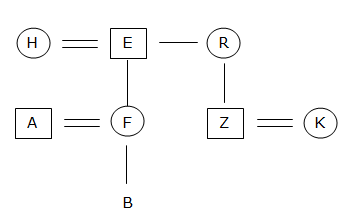1. (d)

2. (b)

D.3-4)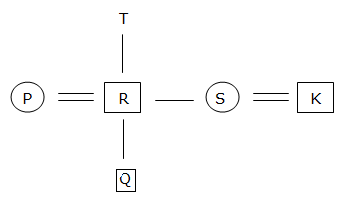3. (c)

4. (a)

5. (b)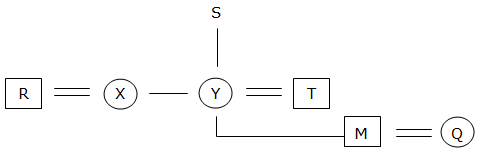D.6-10)

6. (b)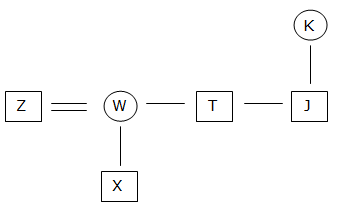7. (a)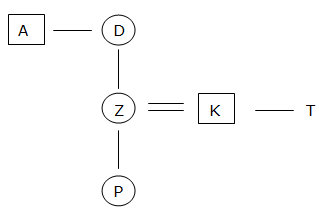8. (d)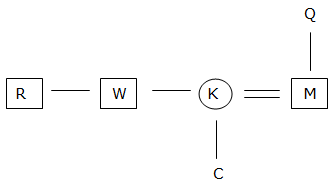9. (d)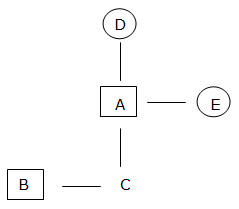10. (d)

You may also like:

TARGET SBI EXAM 2018: REASONING -Alphanumeric series

TARGET SBI EXAM 2018: REASONING –Order & ranking – 1